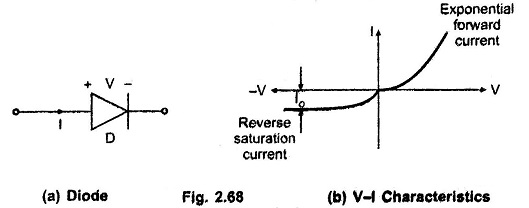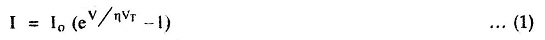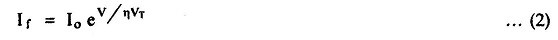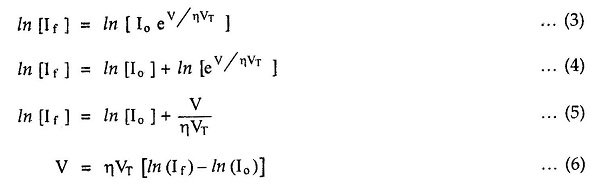## Op Amp with Diode Circuit:

Consider a Op Amp with Diode Circuit as shown in the Fig. 2.68 (a) and the corresponding volt-ampere (V-I) characteristics in the Fig. 2.68 (b).The basic volt-ampere relationship for a diode by using Op amp Circuits is given as,where

• I = diode current
• Io = reverse saturation current
• V = diode voltage
• η = 1 for Ge diode, 2 for Si diode
• VT = kT = voltage equivalent of temperature
• k = Boltzmann’s constant = 8.62 x 10-5 eV/K
• T = temperature in K

It can be observed that at room temperature of 27°C i.e. T = 300°K, the voltage equivalent of temperature is,

VT = 0.02586 V = 26 mV at 300K

This value is frequently used in the diode circuits.

It can be seen from the V-I characteristics shown in the Fig. 2.68 (b) that for a forward biased condition, the diode voltage V is positive and the exponential term eV/ηVT has a positive index. Hence we can say that 1 ≪ eV/ηVT and can be neglected. The corresponding diode current is its forward current If.This indicates that the forward current increases exponentially with respect to the bias voltage V.

Taking natural logarithm of both sides,This equation (6) is the basic equation used in Log and Antilog amplifiers.

Scroll to Top